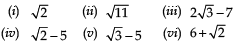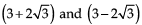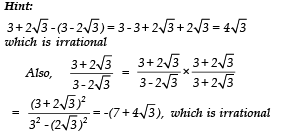Courses

# Worksheet - Real Numbers Class 10 Notes | EduRev

## Class 10 : Worksheet - Real Numbers Class 10 Notes | EduRev

The document Worksheet - Real Numbers Class 10 Notes | EduRev is a part of the Class 10 Course Class 10 Mathematics by VP Classes.
All you need of Class 10 at this link: Class 10

1. Find the H CF of 231 and 396, using Euclid’s division algorithm.

2. Can two numbers have 18 as their HCF and 380 as their LCM? Give reason.

3. Show that the square of an odd positive integer is of the form 8m + 1, for some wholenumber m.

Hint:
For a whole number ‘q’, we have (2q + 1) any positive integer.
∵ (2q + 1)2 = 4q2 + 4q + 1 = 4q(q + 1) + 1
Since, q(q + 1) is either 0 or even
∵ Let it be 2m, where m is a whole number
⇒ (2q + 1)2 = 4[q(q + 1)] + 1
= 4[2m] + 1
= 8m + 1

4. Find the largest number which divides 248 and 1032 leaving remainder 8 in each case.

5. Given that LCM of two numbers is 1449 and their HCF is 23. If one of the numbers is 207, find the other.

6. Find the greatest number of 6-digits which is  exactly divisible by 15, 24 and 36.

7. Prove tha teach of the following is an irrational.8. Prove that p is not a rational number, if p is not a perfect square.

9. Prove that the difference and quotient ofare irrational.ANSWERS
1. 33
2. No, [∵ the HCF (18) does not divide LCM (380)]
4. 16
5. 161
6. 999720

Offer running on EduRev: Apply code STAYHOME200 to get INR 200 off on our premium plan EduRev Infinity!

132 docs

,

,

,

,

,

,

,

,

,

,

,

,

,

,

,

,

,

,

,

,

,

;